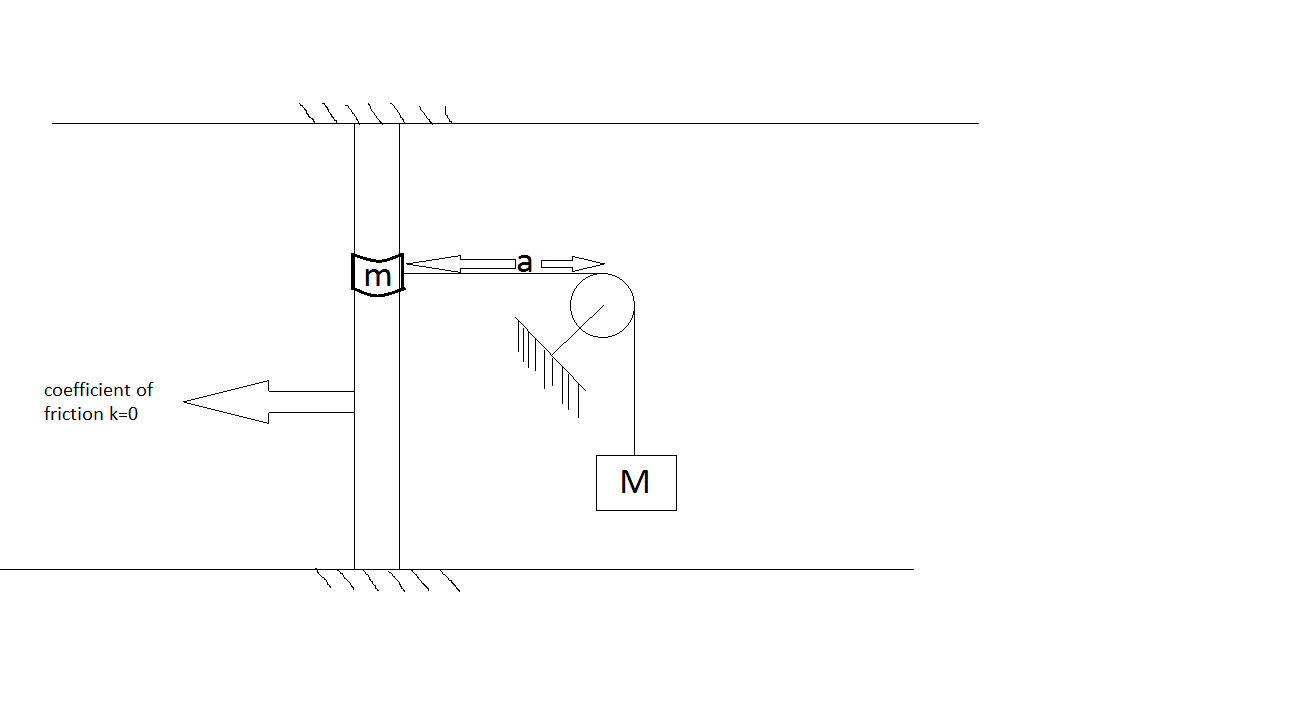# ChallengingA ring of mass $m$ slides on a smooth vertical rod. A light string is attached to the ring and is passing over a smooth pulley over a smooth peg at a distance $a$ from the rod, and at the other end of the string is a mass $M>m$. The ring is held on a level with the peg and released. What is the distance traveled before it comes to rest for first time?

Assumptions and Details

• $M=20$ kg
• $m=10$ kg
• $a=3$ m
×

Problem Loading...

Note Loading...

Set Loading...